# MP Board Class 10th Maths Solutions Chapter 11 Constructions Ex 11.2

In this article, we will share MP Board Class 10th Maths Book Solutions Chapter 11 Constructions Ex 11.2 Pdf, These solutions are solved subject experts from the latest edition books.

## MP Board Class 10th Maths Solutions Chapter 11 Constructions Ex 11.2

In each of the following, give also the justification of the construction:

Question 1.
Draw a circle of radius 6 cm. From a point 10 cm away from its centre, construct the pair of tangents to the circle and measure their lengths.
Solution:
Steps of Construction :
I. Draw a circle of radius 6 cm. Let its centre be O.
II. Take a point P such that OP = 10 cm. Join OP.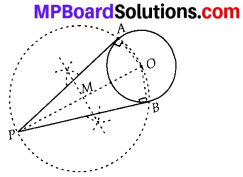III. Bisect OP and let M be its midpoint.
IV. Taking M as centre and MP or MO as radius draw a circle.
Let the new circle intersects the given circle at A and B. Join PA and PB.
Thus, PA and PB are the required tangents. By measurement, we have : PA = PB = 8 cm.
Justification:
Join OA and OB
Since PO is a diameter.
∴ ∠OAP = 90° = ∠OBP [Angles in a semicircle]
Also, OA and OB are radii of the same circle.
⇒ PA and PB are tangents to the circle.

Question 2.
Construct a tangent to a circle of radius 4 cm from a point on the concentric circle of radius 6 cm and measure its length. Also verify the measurement by actual calculation.
Solution:
Steps of Construction :
I. Draw two concentric circles with centre O and radii 4 cm and 6 cm.
II. Take any point P on outer circle.
III. Join PO and bisect it and let the midpoint of PO is represented by M.
IV. Taking M as centre and OM or MP as radius, draw a circle such that this circle intersects the circle (of radius 4 cm) at A and B.
V. Join AP.
Thus, PA is the required tangent.
By measurement, we have PA = 4.5 cm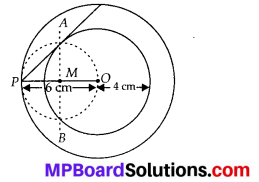Justification:
Join OA. As PO is diameter
∴ ∠PAO = 90° [Angle in a semi-circle]
⇒ PA⊥OA
∵ OA is a radius of the inner circle.
∴ PA has to be a tangent to the inner circle.
Verification:
In right ∆PAO, PO = 6 cm, OA = 4 cm.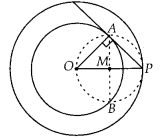Hence both lengths are approximately equal.Question 3.
Draw a circle of radius 3 cm. Take two points P and Q on one of its extended diameter each at a distance of 7 cm from its centre. Draw tangents to the circle from these two points P and Q.
Solution:
Steps of Construction :
I. Draw a circle of radius 3 cm with centre O and draw a diameter.
II. Extend its diameter on both sides and cut OP = OQ = 7 cm
III. Bisect PO such that M be its mid-point.
IV. Taking M as centre and MO as radius, draw a circle. Let it intersect the given circle at A and B.
V. Join PA and PB.
Thus, PA and PB are the two required tangents from P.
VI. Now bisect OQ such that N is its mid point.
VII. Taking N as centre and NO as radius, draw a circle. Let it intersect the given circle at C and D.
VIII. Join QC and QD.
Thus, QC and QD are the required tangents from Question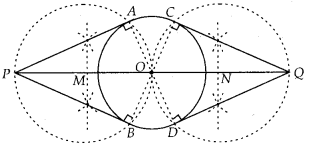Justification:
Join OA to get ∠OAP = 90°
[Angle in a semi-circle]
⇒ PA⊥OA
⇒ PA is a tangent.
Similarly, PB⊥OB
⇒ PB is a tangent.
Now, join OC to get ∠QCO = 90°
[Angle in a semi-circle]
⇒ QC⊥OC ⇒ QC is a tangent.
Similarly, QD⊥OD
⇒ QD is a tangent.

Question 4.
Draw a pair of tangents to a circle of radius 5 cm which are inclined to each other at an angle of 60°.
Solution:
Steps of Construction :
I. With centre O and radius = 5 cm, draw a circle.
II. Taking a point A on the circle draw ∠AOB = 120°.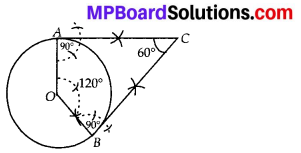III. Draw a perpendicular on OA at A.
IV. Draw another perpendicular on OB at B.
V. Let the two perpendiculars meet at C.
Thus CA and CB are the two required tangents to the given circle which are inclined to each other at 60°.
Justification:
In a quadrilateral OACB, using angle sum
property, we have
120° + 90° + 90° + ∠ACB = 360°
⇒ 300° + ∠ACB = 360°
⇒ ∠ACB = 360° – 300° = 60°.

Question 5.
Draw a line segment AB of length 8 cm. Taking A as centre, draw a circle of radius 4 cm and taking B as centre, draw another circle of radius 3 cm. Construct tangents to each circle from the centre of the other circle.
Solution:
Steps of Construction:
I. Draw a line segment AB = 8 cm
II. Draw a circle with centre A and radius 4 cm, draw another circle with centre B and radius 3 cm.
III. Bisect the line segment AB. Let its mid point be M.
IV. With centre as M and MA (or MB) as radius, draw a circle such that it intersects the two circles at points P, Q, R and S.
V. Join BP and BQ.
Thus, BP and BQ are the required two tangents from B to the circle with centre A.
VI. Join RA and SA.
Thus, RA and SA are the required two tangents from A to the circle with centre B.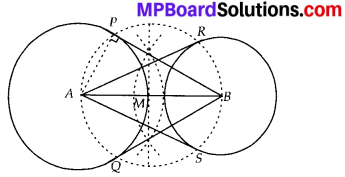Justification:
Let us join A and P.
∵ ∠APB = 90° [Angle in a semi-circle]
∴ BP⊥AP
But AP is radius of the circle with centre A.
⇒ BP has to be a tangent to the circle with centre A.
Similarly, BQ has to be tangent to the circle with centre A.
Also AR and AS are tangents to the circle with centre B.Question 6.
Let ABC be a right triangle in which AB = 6 cm, BC = 8 cm and ∠B = 90°. BD is the perpendicular from B on AC. The circle through B, C, D is drawn. Construct the tangents from A to this circle.
Solution:
Steps of construction :
I. Draw ∆ABC such that AB = 6 cm, BC = 8 cm and ∠B = 90°.
II. Draw BD⊥AC. Now bisect BC and let its midpoint be O.
So O is centre of the circle passing through B, C and D.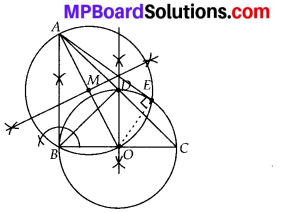III. Join AO
IV. Bisect AO. Let M be the mid-point of AO.
V. Taking M as centre and MA as radius, draw a circle intersecting the given circle at B and E.
VI. Join AB and AE. Thus, AB and AE are the required two tangents to the given circle from A.
Justification:
Join OE, then ∠AEO = 90° [Angle in a semi circle]
∴ AE⊥OE.
But OE is a radius of the given circle.
⇒ AE has to be a tangent to the circle.
Similarly, AB is also a tangent to the given circle.

Question 7.
Draw a circle with the help of a bangle. Take a point outside the circle. Construct the pair of tangents from this point to the circle.
Solution:
Steps of Construction:
I. Draw the given circle using a bangle.
II. Take two non parallel chords PQ and RS of this circle.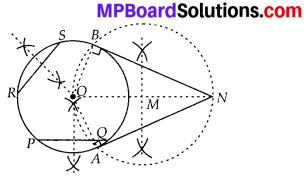III. Draw the perpendicular bisectors of PQ and RS such that they intersect at O. Therefore, O is the centre of the given circle.
IV. Take a point N outside this circle.
V. Join ON and bisect it. Let M be the mid-point of ON.
VI. Taking M as centre and OM as radius, draw a circle. Let it intersect the given circle at A and B.
VII. Join NA and NB. Thus, NA and NB are the required two tangents.
Justification:
Join OA and OB.
Since ∠OAN = 90° [Angle in a semi circle]
∴ NA⊥OA.